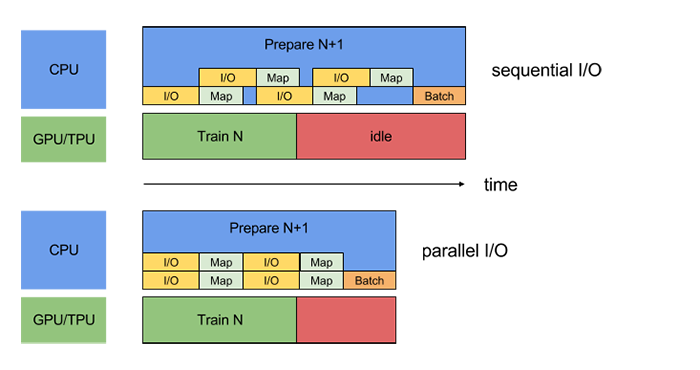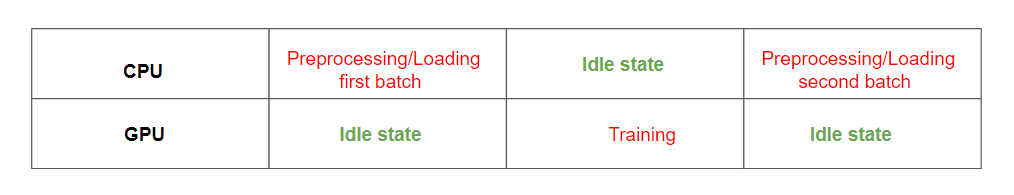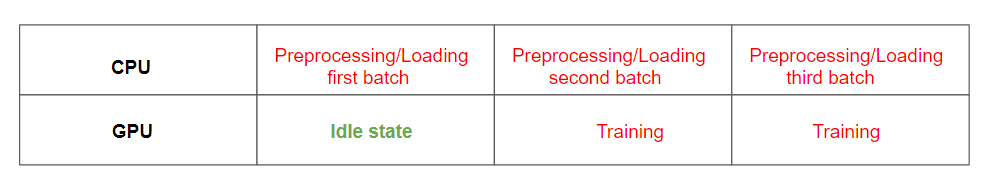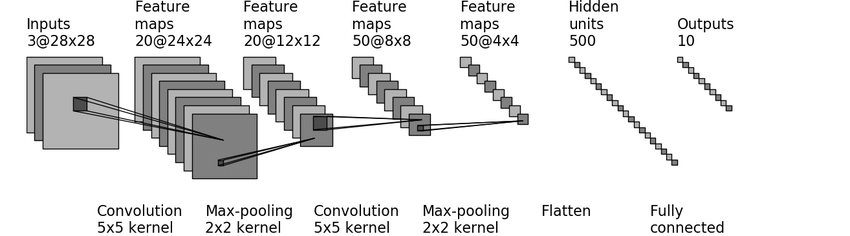1554088939

# Building efficient data pipelines using TensorFlow

Having efficient data pipelines is of paramount importance for any machine learning model. In this blog, we will learn how to use TensorFlow’s Dataset module tf.data to build efficient data pipelines.#### Motivation

Most of the introductory articles on TensorFlow would introduce you with the `feed_dict` method of feeding the data to the model. `feed_dict` processes the input data in a single thread and while the data is being loaded and processed on CPU, the GPU remains idle and when the GPU is training a batch of data, CPU remains in the idle state. The developers of TensorFlow have advised not to use this method during training or repeated validation of the same datasets.`tf_data` improves the performance by prefetching the next batch of data asynchronously so that GPU need not wait for the data. You can also parallelize the process of preprocessing and loading the dataset.In this blog post we will cover `Datasets` and `Iterators`. We will learn how to create Datasets from source data, apply the transformation to Dataset and then consume the data using Iterators.

### How to create Datasets?

Tensorflow provides various methods to create Datasets from numpy arrays, text files, CSV files, tensors, etc. Let’s look at few methods below

• from_tensor_slices: It accepts single or multiple numpy arrays or tensors. Dataset created using this method will emit only one data at a time.
```# source data - numpy array
data = np.arange(10)
# create a dataset from numpy array
dataset = tf.data.Dataset.from_tensor_slices(data)
```

The object `dataset` is a tensorflow Dataset object.

• from_tensors: It also accepts single or multiple numpy arrays or tensors. Dataset created using this method will emit all the data at once.
```data = tf.arange(10)
dataset = tf.data.Dataset.from_tensors(data)
```

3. from_generator: Creates a Dataset whose elements are generated by a function.

```def generator():
for i in range(10):
yield 2*i
dataset = tf.data.Dataset.from_generator(generator, (tf.int32))
```

### Operations on Datasets

• Batches: Combines consecutive elements of the Dataset into a single batch. Useful when you want to train smaller batches of data to avoid out of memory errors.
```data = np.arange(10,40)
create batches of 10
dataset = tf.data.Dataset.from_tensor_slices(data).batch(10)
creates the iterator to consume the data
iterator = dataset.make_one_shot_iterator()
next_ele = iterator.get_next()
with tf.Session() as sess:
try:
while True:
val = sess.run(next_ele)
print(val)
except tf.errors.OutOfRangeError:
pass
```

You can skip the code where we create an iterator and print the elements of the Dataset. We will learn about Iterators in detail in the later part of this blog. The output is :

```[10 11 12 13 14 15 16 17 18 19]
[20 21 22 23 24 25 26 27 28 29]
[30 31 32 33 34 35 36 37 38 39]
```
• Zip: Creates a Dataset by zipping together datasets. Useful in scenarios where you have features and labels and you need to provide the pair of feature and label for training the model.
```datax = np.arange(10,20)
datay = np.arange(11,21)
datasetx = tf.data.Dataset.from_tensor_slices(datax)
datasety = tf.data.Dataset.from_tensor_slices(datay)
dcombined = tf.data.Dataset.zip((datasetx, datasety)).batch(2)
iterator = dcombined.make_one_shot_iterator()
next_ele = iterator.get_next()
with tf.Session() as sess:
try:
while True:
val = sess.run(next_ele)
print(val)
except tf.errors.OutOfRangeError:
pass
```

The output is

```(array([10, 11]), array([11, 12]))
(array([12, 13]), array([13, 14]))
(array([14, 15]), array([15, 16]))
(array([16, 17]), array([17, 18]))
(array([18, 19]), array([19, 20]))
```
• Repeat: Used to repeat the Dataset.
```dataset = tf.data.Dataset.from_tensor_slices(tf.range(10))
dataset = dataset.repeat(count=2)
iterator = dataset.make_one_shot_iterator()
next_ele = iterator.get_next()
with tf.Session() as sess:
try:
while True:
val = sess.run(next_ele)
print(val)
except tf.errors.OutOfRangeError:
pass
```

The output is

```0 1 2 3 4 5 6 7 8 9 0 1 2 3 4 5 6 7 8 9
```
• Map: Used to transform the elements of the Dataset. Useful in cases where you want to transform your raw data before feeding into the model.
```def map_fnc(x):
return x*2;
data = np.arange(10)
dataset = tf.data.Dataset.from_tensor_slices(data)
dataset = dataset.map(map_fnc)
iterator = dataset.make_one_shot_iterator()
next_ele = iterator.get_next()
with tf.Session() as sess:
try:
while True:
val = sess.run(next_ele)
print(val)
except tf.errors.OutOfRangeError:
pass
```

The output is

```0 2 4 6 8 10 12 14 16 18
```

### Creating Iterators

We have learned various ways to create Datasets and apply various transformations to it, but how do we consume the data? Tensorflow provides `Iterators` to do that.

The iterator is not aware of the number of elements present in the Dataset. It has a `get_next` function that is used to create an operation in the tensorflow graph and when run over a session, it will return the values from the iterator. Once the Dataset is exhausted, it throws an `tf.errors.OutOfRangeError` exception.

Let’s look at various `Iterators` that TensorFlow provides.

• One-shot iterator: This is the most basic form of iterator. It requires no explicit initialization and iterates over the data only one time and once it gets exhausted, it cannot be re-initialized.
```data = np.arange(10,15)
#create the dataset
dataset = tf.data.Dataset.from_tensor_slices(data)
#create the iterator
iterator = dataset.make_one_shot_iterator()
next_element = iterator.get_next()
with tf.Session() as sess:
val = sess.run(next_element)
print(val)
```
• Initializable iterator: This iterator requires you to explicitly initialize the iterator by running `iterator.initialize.` You can define a `tf.placeholder` and pass data to it dynamically each time you call the initialize operation.
```# define two placeholders to accept min and max value
min_val = tf.placeholder(tf.int32, shape=[])
max_val = tf.placeholder(tf.int32, shape=[])
data = tf.range(min_val, max_val)
dataset = tf.data.Dataset.from_tensor_slices(data)
iterator = dataset.make_initializable_iterator()
next_ele = iterator.get_next()
with tf.Session() as sess:
# initialize an iterator with range of values from 10 to 15
sess.run(iterator.initializer, feed_dict={min_val:10, max_val:15})
try:
while True:
val = sess.run(next_ele)
print(val)
except tf.errors.OutOfRangeError:
pass
# initialize an iterator with range of values from 1 to 10
sess.run(iterator.initializer, feed_dict={min_val:1, max_val:10})
try:
while True:
val = sess.run(next_ele)
print(val)
except tf.errors.OutOfRangeError:
pass
```
• Reinitializable iterator: This iterator can be initialized from different Dataset objects that have the same structure. Each dataset can pass through its own transformation pipeline.
```def map_fnc(ele):
return ele*2
min_val = tf.placeholder(tf.int32, shape=[])
max_val = tf.placeholder(tf.int32, shape=[])
data = tf.range(min_val, max_val)
#Define separate datasets for training and validation
train_dataset =  tf.data.Dataset.from_tensor_slices(data)
val_dataset = tf.data.Dataset.from_tensor_slices(data).map(map_fnc)
#create an iterator
iterator=tf.data.Iterator.from_structure(train_dataset.output_types    ,train_dataset.output_shapes)
train_initializer = iterator.make_initializer(train_dataset)
val_initializer = iterator.make_initializer(val_dataset)
next_ele = iterator.get_next()
with tf.Session() as sess:
initialize an iterator with range of values from 10 to 15
sess.run(train_initializer, feed_dict={min_val:10, max_val:15})
try:
while True:
val = sess.run(next_ele)
print(val)
except tf.errors.OutOfRangeError:
pass
initialize an iterator with range of values from 1 to 10
sess.run(val_initializer, feed_dict={min_val:1, max_val:10})
try:
while True:
val = sess.run(next_ele)
print(val)
except tf.errors.OutOfRangeError:
pass
```
• Feedable iterator: Can be used to switch between Iterators for different Datasets. Useful when you have different Datasets and you want to have more control over which iterator to use over the Dataset.
```def map_fnc(ele):
return ele*2
min_val = tf.placeholder(tf.int32, shape=[])
max_val = tf.placeholder(tf.int32, shape=[])
data = tf.range(min_val, max_val)
train_dataset = tf.data.Dataset.from_tensor_slices(data)
val_dataset = tf.data.Dataset.from_tensor_slices(data).map(map_fnc)
train_val_iterator = tf.data.Iterator.from_structure(train_dataset.output_types , train_dataset.output_shapes)
train_initializer = train_val_iterator.make_initializer(train_dataset)
val_initializer = train_val_iterator.make_initializer(val_dataset)
test_dataset = tf.data.Dataset.from_tensor_slices(tf.range(10,15))
test_iterator = test_dataset.make_one_shot_iterator()
handle = tf.placeholder(tf.string, shape=[])
iterator = tf.data.Iterator.from_string_handle(handle, train_dataset.output_types, train_dataset.output_shapes)
next_ele = iterator.get_next()
with tf.Session() as sess:
train_val_handle = sess.run(train_val_iterator.string_handle())
test_handle = sess.run(test_iterator.string_handle())
training
sess.run(train_initializer, feed_dict={min_val:10, max_val:15})
try:
while True:
val = sess.run(next_ele, feed_dict={handle:train_val_handle})
print(val)
except tf.errors.OutOfRangeError:
pass
#validation
sess.run(val_initializer, feed_dict={min_val:1, max_val:10})
try:
while True:
val = sess.run(next_ele, feed_dict={handle:train_val_handle})
print(val)
except tf.errors.OutOfRangeError:
pass
#testing
try:
while True:
val = sess.run(next_ele, feed_dict={handle:test_handle})
print(val)
except tf.errors.OutOfRangeError:
pass
```

We have learned about various iterators. Let’s apply the knowledge to a practical dataset. We will train the famous MNIST dataset using the LeNet-5 Model. This tutorial will not dive into the details of implementing the LeNet-5 Model as it is beyond the scope of this article.

### LeNet-5 Model

Let’s import the MNIST data from the tensorflow library. The MNIST database contains 60,000 training images and 10,000 testing images. Each image is of size 28281. We need to resize it to 32321 for the LeNet-5 Model.

```from tensorflow.examples.tutorials.mnist import input_data
mnist = input_data.read_data_sets(“MNIST_data/”, reshape=False, one_hot = True)
X_train, y_train = mnist.train.images, mnist.train.labels
X_val, y_val = mnist.validation.images, mnist.validation.labels
X_test, y_test = mnist.test.images, mnist.test.labels
X_train = np.pad(X_train, ((0,0), (2,2), (2,2), (0,0)), ‘constant’)
X_val =   np.pad(X_val, ((0,0), (2,2), (2,2), (0,0)), ‘constant’)
X_test =  np.pad(X_test, ((0,0), (2,2), (2,2), (0,0)), ‘constant’)
```Let’s define the forward propagation of the model.

```def forward_pass(X):
W1 = tf.get_variable(“W1”, [5,5,1,6], initializer = tf.contrib.layers.xavier_initializer(seed=0))
# for conv layer2
W2 = tf.get_variable(“W2”, [5,5,6,16], initializer = tf.contrib.layers.xavier_initializer(seed=0))
Z1 = tf.nn.conv2d(X, W1, strides = [1,1,1,1], padding=‘VALID’)
A1 = tf.nn.relu(Z1)
P1 = tf.nn.max_pool(A1, ksize = [1,2,2,1], strides = [1,2,2,1], padding=‘VALID’)
Z2 = tf.nn.conv2d(P1, W2, strides = [1,1,1,1], padding=‘VALID’)
A2= tf.nn.relu(Z2)
P2= tf.nn.max_pool(A2, ksize = [1,2,2,1], strides=[1,2,2,1], padding=‘VALID’)
P2 = tf.contrib.layers.flatten(P2)
```Z3 = tf.contrib.layers.fully_connected(P2, 120)
Z4 = tf.contrib.layers.fully_connected(Z3, 84)
Z5 = tf.contrib.layers.fully_connected(Z4,10, activation_fn= None)
return Z5
```
```

Let’s define the model operations

```def model(X,Y):
```logits = forward_pass(X)
cost = tf.reduce_mean( tf.nn.softmax_cross_entropy_with_logits_v2(logits=logits, labels=Y))
learner = optimizer.minimize(cost)
correct_predictions = tf.equal(tf.argmax(logits,1),   tf.argmax(Y,1))
accuracy = tf.reduce_mean(tf.cast(correct_predictions, tf.float32))

return (learner, accuracy)
```
```

We have now created the model. Before deciding on the Iterator to use for our model, let’s see what are the typical requirements of a machine learning model.

1. Training the data over batches: Dataset can be very huge. To prevent out of memory errors, we would need to train our dataset in small batches.
2. Train the model over n passes of the dataset: Typically you want to run your training model over multiple passes of the dataset.
3. Validate the model at each epoch: You would need to validate your model at each epoch to check your model’s performance.
4. Finally, test your model on unseen data: After the model is trained, you would like to test your model on unseen data.

Let’s see the pros and cons of each iterator.

• One-shot iterator: The Dataset can’t be reinitialized once exhausted. To train for more epochs, you would need to repeat the Dataset before feeding to the iterator. This will require huge memory if the size of the data is large. It also doesn’t provide any option to validate the model.
```epochs = 10
batch_size = 64
iterations = len(y_train) * epochs
tf.reset_default_graph()
dataset = tf.data.Dataset.from_tensor_slices((X_train, y_train))
need to repeat the dataset for epoch number of times, as all the data needs
to be fed to the dataset at once
dataset = dataset.repeat(epochs).batch(batch_size)
iterator = dataset.make_one_shot_iterator()
X_batch , Y_batch = iterator.get_next()
(learner, accuracy) = model(X_batch, Y_batch)
with tf.Session() as sess:
sess.run(tf.global_variables_initializer())
total_accuracy = 0
try:
while True:
temp_accuracy, _ = sess.run([accuracy, learner])
total_accuracy += temp_accuracy
except tf.errors.OutOfRangeError:
pass
print(‘Avg training accuracy is {}’.format((total_accuracy * batch_size) / iterations ))
```
• Initializable iterator: You can dynamically change the Dataset between training and validation Datasets. However, in this case both the Datasets needs to go through the same transformation pipeline.
```epochs = 10
batch_size = 64
tf.reset_default_graph()
X_data = tf.placeholder(tf.float32, [None, 32,32,1])
Y_data = tf.placeholder(tf.float32, [None, 10])
dataset = tf.data.Dataset.from_tensor_slices((X_data, Y_data))
dataset = dataset.batch(batch_size)
iterator = dataset.make_initializable_iterator()
X_batch , Y_batch = iterator.get_next()
(learner, accuracy) = model(X_batch, Y_batch)
with tf.Session() as sess:
sess.run(tf.global_variables_initializer())
for epoch in range(epochs):
```# train the model
sess.run(iterator.initializer, feed_dict={X_data:X_train, Y_data:y_train})
total_train_accuracy = 0
no_train_examples = len(y_train)
try:
while True:
temp_train_accuracy, _ = sess.run([accuracy, learner])
total_train_accuracy += temp_train_accuracy*batch_size
except tf.errors.OutOfRangeError:
pass

# validate the model
sess.run(iterator.initializer, feed_dict={X_data:X_val, Y_data:y_val})
total_val_accuracy = 0
no_val_examples = len(y_val)
try:
while True:
temp_val_accuracy = sess.run(accuracy)
total_val_accuracy += temp_val_accuracy*batch_size
except tf.errors.OutOfRangeError:
pass

print('Epoch {}'.format(str(epoch+1)))
print("---------------------------")
print('Training accuracy is {}'.format(total_train_accuracy/no_train_examples))
print('Validation accuracy is {}'.format(total_val_accuracy/no_val_examples))
```
```
• Re-initializable iterator: This iterator overcomes the problem of initializable iterator by using two separate Datasets. Each dataset can go through its own preprocessing pipeline. The iterator can be created using the `tf.Iterator.from_structure` method.
```def map_fnc(X, Y):
return X, Y
epochs = 10
batch_size = 64
tf.reset_default_graph()
X_data = tf.placeholder(tf.float32, [None, 32,32,1])
Y_data = tf.placeholder(tf.float32, [None, 10])
train_dataset = tf.data.Dataset.from_tensor_slices((X_data, Y_data)).batch(batch_size).map(map_fnc)
val_dataset =  tf.data.Dataset.from_tensor_slices((X_data, Y_data)).batch(batch_size)
iterator = tf.data.Iterator.from_structure(train_dataset.output_types, train_dataset.output_shapes)
X_batch , Y_batch = iterator.get_next()
(learner, accuracy) = model(X_batch, Y_batch)
train_initializer = iterator.make_initializer(train_dataset)
val_initializer =  iterator.make_initializer(val_dataset)
with tf.Session() as sess:
sess.run(tf.global_variables_initializer())
for epoch in range(epochs):
```# train the model
sess.run(train_initializer, feed_dict={X_data:X_train, Y_data:y_train})
total_train_accuracy = 0
no_train_examples = len(y_train)
try:
while True:
temp_train_accuracy, _ = sess.run([accuracy, learner])
total_train_accuracy += temp_train_accuracy*batch_size
except tf.errors.OutOfRangeError:
pass

# validate the model
sess.run(val_initializer, feed_dict={X_data:X_val, Y_data:y_val})
total_val_accuracy = 0
no_val_examples = len(y_val)
try:
while True:
temp_val_accuracy = sess.run(accuracy)
total_val_accuracy += temp_val_accuracy*batch_size
except tf.errors.OutOfRangeError:
pass

print('Epoch {}'.format(str(epoch+1)))
print("---------------------------")
print('Training accuracy is {}'.format(total_train_accuracy/no_train_examples))
print('Validation accuracy is {}'.format(total_val_accuracy/no_val_examples))
```
```
• Feedable iterator: This iterator provides the option of switching between various iterators. You can create a re-initializable iterator for training and validation purposes. For inference/testing where you require one pass of the dataset, you can use the one shot iterator.
```epochs = 10
batch_size = 64
tf.reset_default_graph()
X_data = tf.placeholder(tf.float32, [None, 32,32,1])
Y_data = tf.placeholder(tf.float32, [None, 10])
train_dataset = tf.data.Dataset.from_tensor_slices((X_data, Y_data)).batch(batch_size)
val_dataset =  tf.data.Dataset.from_tensor_slices((X_data, Y_data)).batch(batch_size)
test_dataset =  tf.data.Dataset.from_tensor_slices((X_test, y_test.astype(np.float32)).batch(batch_size)
handle = tf.placeholder(tf.string, shape=[])
iterator = tf.data.Iterator.from_string_handle(handle, train_dataset.output_types, train_dataset.output_shapes)
X_batch , Y_batch = iterator.get_next()
(learner, accuracy) = model(X_batch, Y_batch)
train_val_iterator = tf.data.Iterator.from_structure(train_dataset.output_types, train_dataset.output_shapes)
train_iterator = train_val_iterator.make_initializer(train_dataset)
val_iterator = train_val_iterator.make_initializer(val_dataset)
test_iterator = test_dataset.make_one_shot_iterator()
with tf.Session() as sess:
sess.run(tf.global_variables_initializer())
train_val_string_handle = sess.run(train_val_iterator.string_handle())
test_string_handle = sess.run(test_iterator.string_handle())
for epoch in range(epochs):
```# train the model
sess.run(train_iterator, feed_dict={X_data:X_train, Y_data:y_train})
total_train_accuracy = 0
no_train_examples = len(y_train)
try:
while True:
temp_train_accuracy, _ = sess.run([accuracy, learner], feed_dict={handle:train_val_string_handle})
total_train_accuracy += temp_train_accuracy*batch_size
except tf.errors.OutOfRangeError:
pass

# validate the model
sess.run(val_iterator, feed_dict={X_data:X_val, Y_data:y_val})
total_val_accuracy = 0
no_val_examples = len(y_val)
try:
while True:
temp_val_accuracy, _ = sess.run([accuracy, learner], feed_dict={handle:train_val_string_handle})
total_val_accuracy += temp_val_accuracy*batch_size
except tf.errors.OutOfRangeError:
pass

print('Epoch {}'.format(str(epoch+1)))
print("---------------------------")
print('Training accuracy is {}'.format(total_train_accuracy/no_train_examples))
print('Validation accuracy is {}'.format(total_val_accuracy/no_val_examples))
```
print(“Testing the model --------”)
total_test_accuracy = 0
no_test_examples = len(y_test)
try:
while True:
temp_test_accuracy, _ = sess.run([accuracy, learner], feed_dict={handle:test_string_handle})
total_test_accuracy += temp_test_accuracy*batch_size
except tf.errors.OutOfRangeError:
pass
print(‘Testing accuracy is {}’.format(total_test_accuracy/no_test_examples))
```

Thanks for reading the blog. The code examples used in this blog can be found in this jupyter notebook.

Do leave your comments below if you have any questions or if you have any suggestions for improving this blog.

Originally published by Animesh Agarwal at https://towardsdatascience.com/

#tensorflow #data-science

## Buddha Community1620466520

## Your Data Architecture: Simple Best Practices for Your Data Strategy

If you accumulate data on which you base your decision-making as an organization, you should probably think about your data architecture and possible best practices.

If you accumulate data on which you base your decision-making as an organization, you most probably need to think about your data architecture and consider possible best practices. Gaining a competitive edge, remaining customer-centric to the greatest extent possible, and streamlining processes to get on-the-button outcomes can all be traced back to an organization’s capacity to build a future-ready data architecture.

In what follows, we offer a short overview of the overarching capabilities of data architecture. These include user-centricity, elasticity, robustness, and the capacity to ensure the seamless flow of data at all times. Added to these are automation enablement, plus security and data governance considerations. These points from our checklist for what we perceive to be an anticipatory analytics ecosystem.

#big data #data science #big data analytics #data analysis #data architecture #data transformation #data platform #data strategy #cloud data platform #data acquisition1620629020

## Getting Started With Data Lakes

### Frameworks for Efficient Enterprise Analytics

The opportunities big data offers also come with very real challenges that many organizations are facing today. Often, it’s finding the most cost-effective, scalable way to store and process boundless volumes of data in multiple formats that come from a growing number of sources. Then organizations need the analytical capabilities and flexibility to turn this data into insights that can meet their specific business objectives.

This Refcard dives into how a data lake helps tackle these challenges at both ends — from its enhanced architecture that’s designed for efficient data ingestion, storage, and management to its advanced analytics functionality and performance flexibility. You’ll also explore key benefits and common use cases.

### Introduction

As technology continues to evolve with new data sources, such as IoT sensors and social media churning out large volumes of data, there has never been a better time to discuss the possibilities and challenges of managing such data for varying analytical insights. In this Refcard, we dig deep into how data lakes solve the problem of storing and processing enormous amounts of data. While doing so, we also explore the benefits of data lakes, their use cases, and how they differ from data warehouses (DWHs).

This is a preview of the Getting Started With Data Lakes Refcard. To read the entire Refcard, please download the PDF from the link above.

#big data #data analytics #data analysis #business analytics #data warehouse #data storage #data lake #data lake architecture #data lake governance #data lake management1615970748

## Create Efficient Data Pipelines with TFDS and tf.Data

Let’s get these clear, existing Datasets aren’t enough
While most Machine Learning programmers start with common open-source datasets like MNIST or CIFAR-10, and that’s all well and good, but to expand your horizon, and solve problems, you need to go beyond these and get your own data. While the collection of data may or may not be too hard, most people find difficulties in making this data ready for training. This is mostly because of the large number of intermediate steps like format conversion (usually for Computer Vision), Tokenizing (for NLP) and the general steps of Data Augmentation, Shuffling etc.
To make this process easier, let’s first understand this process of getting a Dataset ready.

#data #keras #data-pipeline #tensorflow #tensorflow-dataset1624546800

## Data Lake and Data Mesh Use Cases

As data mesh advocates come to suggest that the data mesh should replace the monolithic, centralized data lake, I wanted to check in with Dipti Borkar, co-founder and Chief Product Officer at Ahana. Dipti has been a tremendous resource for me over the years as she has held leadership positions at Couchbase, Kinetica, and Alluxio.

#### Definitions

• A data lake is a concept consisting of a collection of storage instances of various data assets. These assets are stored in a near-exact, or even exact, copy of the resource format and in addition to the originating data stores.
• A data mesh is a type of data platform architecture that embraces the ubiquity of data in the enterprise by leveraging a domain-oriented, self-serve design. Mesh is an abstraction layer that sits atop data sources and provides access.

According to Dipti, while data lakes and data mesh both have use cases they work well for, data mesh can’t replace the data lake unless all data sources are created equal — and for many, that’s not the case.

#### Data Sources

All data sources are not equal. There are different dimensions of data:

• Amount of data being stored
• Importance of the data
• Type of data
• Type of analysis to be supported
• Longevity of the data being stored
• Cost of managing and processing the data

Each data source has its purpose. Some are built for fast access for small amounts of data, some are meant for real transactions, some are meant for data that applications need, and some are meant for getting insights on large amounts of data.

#### AWS S3

Things changed when AWS commoditized the storage layer with the AWS S3 object-store 15 years ago. Given the ubiquity and affordability of S3 and other cloud storage, companies are moving most of this data to cloud object stores and building data lakes, where it can be analyzed in many different ways.

Because of the low cost, enterprises can store all of their data — enterprise, third-party, IoT, and streaming — into an S3 data lake. However, the data cannot be processed there. You need engines on top like Hive, Presto, and Spark to process it. Hadoop tried to do this with limited success. Presto and Spark have solved the SQL in S3 query problem.

#big data #big data analytics #data lake #data lake and data mesh #data lake #data mesh1618039260

## How Has COVID-19 Impacted Data Science?

The COVID-19 pandemic disrupted supply chains and brought economies around the world to a standstill. In turn, businesses need access to accurate, timely data more than ever before. As a result, the demand for data analytics is skyrocketing as businesses try to navigate an uncertain future. However, the sudden surge in demand comes with its own set of challenges.

Here is how the COVID-19 pandemic is affecting the data industry and how enterprises can prepare for the data challenges to come in 2021 and beyond.

#big data #data #data analysis #data security #data integration #etl #data warehouse #data breach #elt